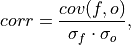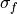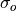# climpred.metrics._pearson_r#

climpred.metrics._pearson_r(forecast: xarray.Dataset, verif: xarray.Dataset, dim: Optional[Union[str, List[str]]] = None, **metric_kwargs: Any) [source]#

Pearson product-moment correlation coefficient.

A measure of the linear association between the forecast and verification data that is independent of the mean and variance of the individual distributions. This is also known as the Anomaly Correlation Coefficient (ACC) when correlating anomalies.whereandrepresent the standard deviation of the forecast and verification data over the experimental period, respectively.

Note

Use metric _pearson_r_p_value() or _pearson_r_eff_p_value() to get the corresponding p value.

Parameters

Notes

 minimum -1.0 maximum 1.0 perfect 1.0 orientation positive

Example

>>> HindcastEnsemble.verify(
...     metric="pearson_r",
...     comparison="e2o",
...     alignment="same_verifs",
...     dim=["init"],
... )
<xarray.Dataset>
Coordinates:
* lead     (lead) int32 1 2 3 4 5 6 7 8 9 10
skill    <U11 'initialized'
Data variables:
SST      (lead) float64 0.9272 0.9145 0.9127 0.9319 ... 0.9315 0.9185 0.9112
Attributes: